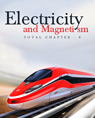Click to Chat

1800-1023-196

+91-120-4616500

CART 0

• 0

MY CART (5)

Use Coupon: CART20 and get 20% off on all online Study Material

ITEM
DETAILS
MRP
DISCOUNT
FINAL PRICE
Total Price: Rs.

There are no items in this cart.
Continue Shopping
`        A capacitor of capacitance C is connected to a battery of emf ℰ at t = 0 through a resistance R. Find the maximum rate at which energy is stored in the capacitor. When does the rate has this maximum value?`
5 years ago

```							Sol. let at any time t, q = EC (1 – e^-t/CR)
E = Energy stored = q^2/2c = E^2C^2/2c (1 – e^-t/CR)^2 = E^2C/2 (1 – e^-t/CR)^2
R = rate of energy stored = dE/dt = -E^2C/2 (-1/RC)^2 (1 – e^-t/RC)e^-t/RC = E^2/CR . e^-t/RC (1 – e^-t/CR) dR/dt = E^2/2R [-1/RC e^-t/CR . (1 – e^-t/CR)+(-) . e^-t/CR(1-/ RC) . e^-t/CR]
E^2/2R = (-e^-t/CR/RC + e^-2t/CR/RC + 1/RC. E^-2t/CR) = E^2/2R(2/RC . e^-2t/CR – e^-t/CR/RC)  ….(1)
For R base max dR/dt = 0 ⇒ 2.e^-t/RC -1 = 0 ⇒ e^-t/CR = ½
⇒ -t/RC = -In^2 ⇒ t = RC In 2
∴ Putting t = RC In 2 in equation (1) We get dR/dt = E^2/4R .

```
5 years ago
Think You Can Provide A Better Answer ?

## Other Related Questions on Electromagnetic Induction

View all Questions »### Course Features

• 731 Video Lectures
• Revision Notes
• Previous Year Papers
• Mind Map
• Study Planner
• NCERT Solutions
• Discussion Forum
• Test paper with Video Solution### Course Features

• 57 Video Lectures
• Revision Notes
• Test paper with Video Solution
• Mind Map
• Study Planner
• NCERT Solutions
• Discussion Forum
• Previous Year Exam Questions